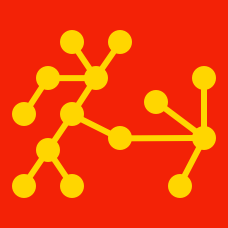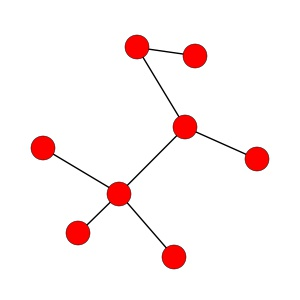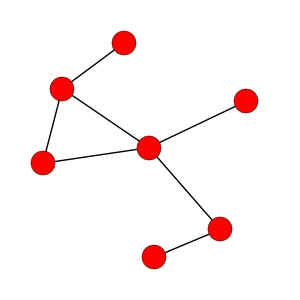Probability

# Trees

True or false: any tree is connected.

A tree has 10 vertices. How many different possibilities are there for the number of edges that it can have?Is this graph a tree?

Define the distance between two nodes to be the number of edges in the shortest path between them. Define the width of a tree to be the largest distance between any two nodes. What's the smallest possible width of a tree with 63 nodes?Is this graph a tree?

×